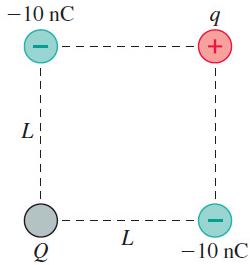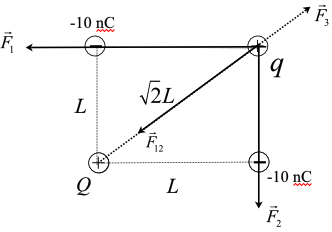In: Physics

# What magnitude and sign of charge Q will make the force on charge q zero?

(Figure 1) shows four charges at the corners of a square of side L.What magnitude and sign of charge Q will make the force on charge q zero?

Q =

## Solution

##### Step #1

The following figure shows the schematic diagram of the arrangement of charges at the vertices of the square of side $$L$$In the above figure $$\vec{F}_{1}$$ is the force on charge $$q$$ due to $$-10 \mathrm{nC}$$ charge, $$\vec{F}_{2}$$ is the force on

charge $$q$$ due to the charge $$-10 \mathrm{nC}, \vec{F}_{3}$$ is the force on charge $$q$$ due to the charge $$Q,$$ and $$\vec{F}_{12}$$ is the net force of $$\vec{F}_{1}$$ and $$\vec{F}_{2}$$

As the charge $$-10 \mathrm{nC}$$ pulls the charge $$q$$ in the directions shown in the figure. The equilibrium is possible only when the sign of charge $$q$$ is positive.

since $$\vec{F}_{1}$$ and $$\vec{F}_{2}$$ are mutually perpendicular,

$$F_{12}=\sqrt{F_{1}^{2}+F_{2}^{2}}$$

The magnitude of force between the charges $$-10 \mathrm{nC}$$ and $$q$$ is, $$\begin{array}{l} F_{1}=k \frac{(-10 \mathrm{n} \mathrm{C}) q}{L^{2}} \\ F_{2}=k \frac{(-10 \mathrm{n} \mathrm{C}) q}{L^{2}} \\ F_{12}=\sqrt{\left(k \frac{(-10 \mathrm{n} \mathrm{C}) q}{L^{2}}\right)^{2}+\left(k \frac{(-10 \mathrm{n} \mathrm{C}) q}{L^{2}}\right)^{2}} \\ F_{12}=\sqrt{2}\left(k \frac{(-10 \mathrm{n} \mathrm{C}) q}{L^{2}}\right) \end{array}$$ The magnitude of force between the charges $$Q$$ and $$q$$ is, $$\begin{array}{l} F_{3}=k \frac{Q q}{(\sqrt{2} L)^{2}} \\ F_{3}=k \frac{Q q}{2 L^{2}} \end{array}$$ From the figure, the forces $$F_{3}$$ and $$F_{12}$$ are in opposite direction and hence, \begin{aligned} k \frac{Q q}{2 L^{2}} &=\sqrt{2}\left(k \frac{(-10 \mathrm{n} \mathrm{C}) q}{L^{2}}\right) \\ \frac{Q}{2} &=\sqrt{2}(-10 \mathrm{n} \mathrm{C}) \\ Q &=28.3 \mathrm{n} \mathrm{C} \end{aligned} Thus, the magnitude of charge is $$28.3 \mathrm{nC}$$.